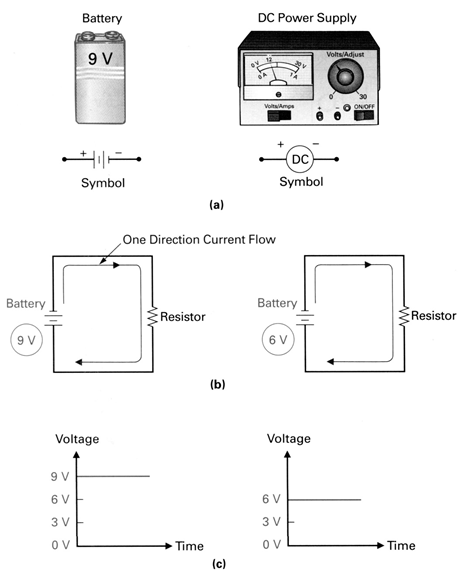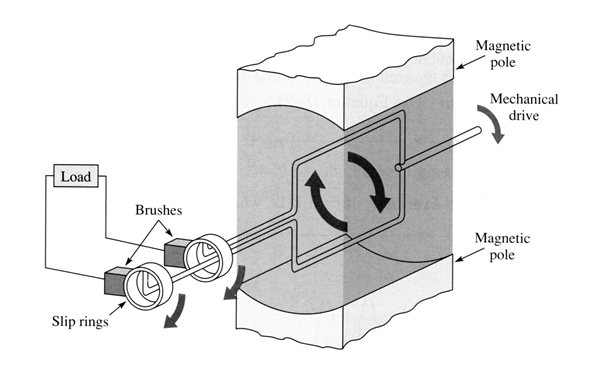Science

# Alternating Current & Direct Current

Mains electricity in the UK is an alternating current (AC) supply (230V). But what is AC and how does it differ to direct current (DC)?

AC produces voltages with alternating polarity, moving forwards and backwards in a circuit over time, either through voltage switching polarity or a current changing direction and back and forth. It appears in a variety of electrical sources, most notably rotary electro-mechanical generators. An alternating current rises to a maximum value in one direction and then falls back to zero, before repeating the process in the opposite direction.

The amount of time it takes for an AC current to rise from zero, reach its peak, return to zero and repeat the process in the opposite direction is called one cycle. The number of cycles occurring every second is called the frequency.

In the UK electricity is supplied as alternating current at a frequency of fifty cycles per second, or 50 hertz (Hz).

## What is the difference between AC and DC?

Where AC reverses polarity back and forth, direct current or DC is electricity that flows in one constant direction around a circuit, and/or has a voltage with constant polarity.

For large current applications, DC generators are needed, where the generator converts mechanical rotation to electrical output. AC generators are less complex and cheaper to operate.

AC is easier to transport over long distances with minimum power loss. Transformers can be used to change voltage, and AC can easily be converted to DC (but DC cannot easily be converted to AC).

## Examples of DC vs AC operations

### DC Operation:### AC Operation:AC voltage waveform constantly changes in magnitude and periodically changes direction. In the diagram below (a) varies around a reference voltage and (b) is usually 0 volts but not necessarily always so.

## What is voltage generation?

When there is a relative motion between a conductor and a magnetic field whereby the flux lines are cut, a voltage is induced into the conductor. The magnitude of that voltage is dependent on the speed of movement.

Either the conductor or a magnetic field can be moved, as long as there is relative motion between the two the voltage is induced.

The amount of voltage induced is dependent on the number of flux lines cut in a specific period. The faster the motion, the more lines cut, consequently a larger voltage is induced. Completing a circuit between the ends of the conductor allows the current to flow.

The polarity of induced voltage depends on the direction of relative motion between the conductor and the magnetic field. When relative motion is parallel to the magnetic field, no voltage is induced. This is because there is no relative motion and so no flux lines are being cut.This is the simplest form of an AC generator. A loop of wire is rotated in a magnetic field, and as the loop is rotated a voltage is induced as it cuts the flux lines. Slip rings pick off induced voltage to cause current to flow through the load.As the loop rotates through points A and C (below) it is parallel to the flux lines so no voltage is induced. As it moves through points B and D it is perpendicular to the flux lines. At this point, the maximum voltage is induced.